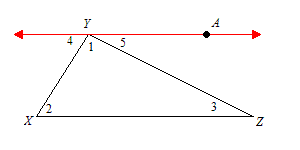# Angle Sum Theorem

The Angle Sum Theorem gives an important result about triangles, which is used in many algebra and geometry problems. We give the proof below.

Theorem: The sum of the measures of the interior angles of a triangle is $180°$.

Given: $\Delta XYZ$

Prove: $m\angle 1+m\angle 2+m\angle 3=180°$Statements Reasons Construct a line parallel to $\overline{XZ}$ through point $Y$. Call this line $\stackrel{↔}{AY}$ Construction $m\angle 1+m\angle 5=m\angle AYX$ Angle Addition Postulate $m\angle AYX+m\angle 4=180°$ Linear Pair Postulate $m\angle 1+m\angle 5+m\angle 4=180°$ Substitution $\begin{array}{l}\angle 2\cong \angle 4\\ \angle 3\cong \angle 5\end{array}$ Alternate Interior Angles Theorem $\begin{array}{l}m\angle 2=m\angle 4\\ m\angle 3=m\angle 5\end{array}$ Definition of Congruence $m\angle 1+m\angle 3+m\angle 2=180°$ Substitution